GFUN AND THE AGM

Bruno Salvy

January 1998

Let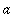and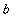be two positive real numbers, with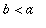. The arithmetic-geometric mean ofandis classically defined as the common limit of the sequences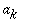and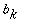defined by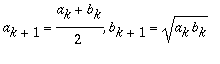, with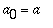and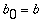.
That the sequences converge to the same limit can be inferred from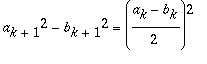.

This common limit is known by Maple as GaussAGM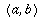. It was discovered by Gauss that the arithmetic-geometric mean is related to hypergeometric functions by

> GaussAGM(a,b)=a/hypergeom([1/2, 1/2],,1-b^2/a^2);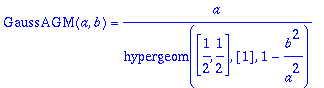> eval(subs(a=3.,b=2.,"));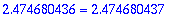This worksheet, largely inspired by , shows how gfun can be used to guess and then prove this result, as well as a generalization of it due to J. M. Borwein and P. B. Borwein.

The functional equation

Following , we start by introducing a generalization of the arithmetic-geometric mean obtained by considering the following iteration where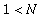is an integer: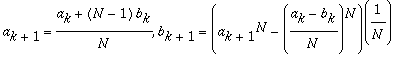.

where the second equality is motivated by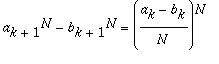from which follows that both sequences converge to a common limit, which is denoted by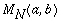. The arithmetic-geometric mean corresponds to the case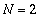.

The functionis easily seen to be homogeneous: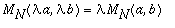, for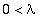. Together with the obvious property that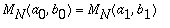, this implies that for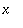in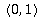,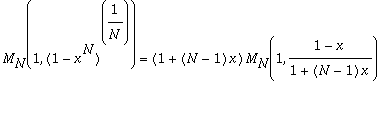.

Defining the function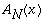by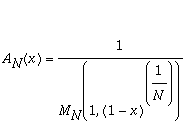the equation above translates into the following functional equation for:

>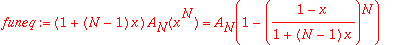which plays a central rôle in this worksheet. It is not too difficult to show thatis analytic in the neighborhood of the origin and that the functional equation above has a unique analytic solution in this neighborhood.

The quadratic case

This is the caseand Gauss's theorem is equivalent to stating that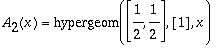.

We now use gfun to first guess and then prove this result. The first step is to use the functional equation to compute a series expansion of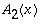, then we use this series to guess a possible closed form which turns out to be analytic, then we show that this analytic function does satisfy the functional equation.

Series expansion

Starting from the functional equation,

> funeq2:=subs(N=2,A=A,op(1,funeq)-op(2,funeq));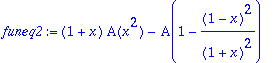a series solution is easily obtained by a method of undeterminate coefficients:

> sol:=1:

> for i to 12 do
sol:=sol+x^i*solve(op(1,series(eval(
subs(A=unapply(sol+a*x^i,x),funeq2)),x,i+2)),a) od:sol;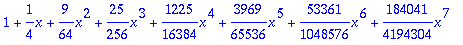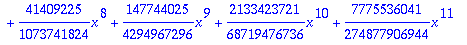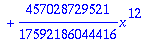Guessing the solution

From this series, gfun guesses a differential equation which could be satisfied by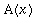:

> deq:=op(1,gfun[seriestodiffeq](series(sol,x,13),y(x),[ogf]));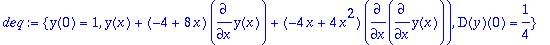It turns out that Maple's dsolve function is unable to solve this differential equation:

> dsolve(deq,y(x));

We then use gfun[diffeqtorec] which deduces from this differential equation the recurrence satisfied by the Taylor coefficients of its solutions:

> gfun[diffeqtorec](deq,y(x),u(n));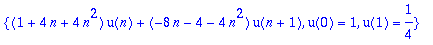From this first order linear recurrence, a solution is easily found:

> rsolve(",u(n));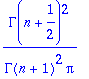hence the sum:

> y(x)=sum("*x^n,n=0..infinity);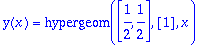Proving the result of the guess

The proof consists in showing that the function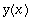, which is obviously analytic, satisfies the functional equation

> subs(A=y,funeq2)=0;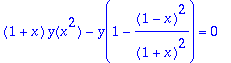Our approach consists in using closure properties of solutions of linear differential equations that are implemented in gfun to compute a linear differential equation satisfied by the left-hand side of this equation. The proof then reduces to showing that 0 is the only solution of this differential equation that is compatible with the initial conditions, which are 0 up to a large order by construction of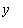.
It turns out that this proof can be performed directly from the differential equation, and would apply even if no closed-form had been found.

Given a linear differential equation satisfied by a series, the function gfun[algebraicsubs] computes a linear differential equation satisfied by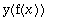for any algebraic function, given by a polynomial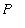such that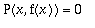. Thus a differential equation satisfied by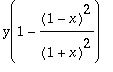is easily computed from that satisfied by:

> deq:=op(select(has,deq,x)):

> deq1:=gfun[algebraicsubs](deq,numer(y-(1-(1-x)^2/(1+x)^2)),y(x));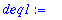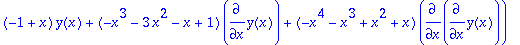Similarly,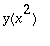satisfies

> gfun[algebraicsubs](deq,y-x^2,y(x));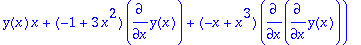and its product by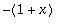satisfies

> deq2:=gfun[`diffeq*diffeq`](",y(x)+1+x,y(x));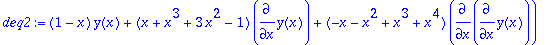From there, we deduce a differential equation satisfied by the left-hand side of the functional equation when applied to the hypergeometric function we have guessed:

> gfun[`diffeq+diffeq`](deq1,deq2,y(x));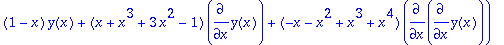Analytic solutions of this equation have a coefficient sequence which satisfies

> gfun[diffeqtorec](",y(x),u(n));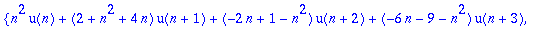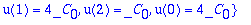and thus the first three zeroes of the Taylor expansion of the left-hand side of the functional equation conclude the proof.

The cubic case

It has been discovered by J. M. Borwein and P. B. Borwein that a hypergeometric expression also exists when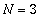. Again, the same steps as above lead to guessing and then proving the following result by gfun .

Theorem . [Borwein & Borwein 90] The function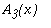corresponding to the AGM iteration of order 3 has the following closed form: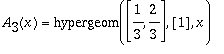.

Series expansion

We start from the functional equation

> funeq3:=subs(N=3,A=A,op(1,funeq)-op(2,funeq));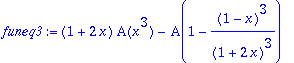and compute the first terms of the series expansion of the solution:

> sol:=1:

> for i to 12 do
sol:=sol+x^i*solve(op(1,series(eval(
subs(A=unapply(sol+a*x^i,x),funeq3)),x,i+2)),a) od:sol;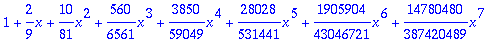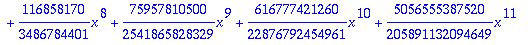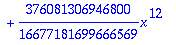Guessing the solution

Again, this is a lucky situation where a differential equation can be guessed:

> deq:=op(1,gfun[seriestodiffeq](series(sol,x,13),y(x),[ogf]));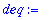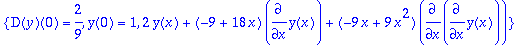From there, we find a closed-form as before

> gfun[diffeqtorec](deq,y(x),u(n));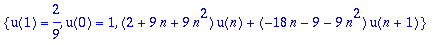> rsolve(",u(n));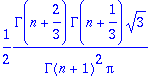> y(x)=sum("*x^n,n=0..infinity);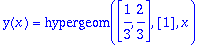Proving the result of the guess

The same routine applies:

> subs(A=y,funeq3)=0;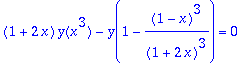> deq:=op(select(has,deq,x)):

> deq1:=gfun[algebraicsubs](deq,numer(y-(1-(1-x)^3/(1+2*x)^3)),y(x));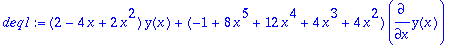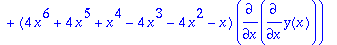> gfun[algebraicsubs](deq,y-x^3,y(x));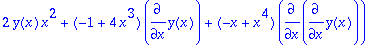> deq2:=gfun[`diffeq*diffeq`](",y(x)+1+2*x,y(x));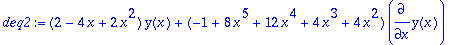> gfun[`diffeq+diffeq`](deq1,deq2,y(x));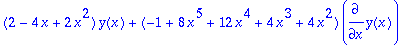> gfun[diffeqtorec](",y(x),u(n));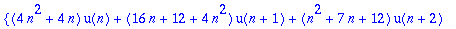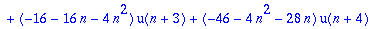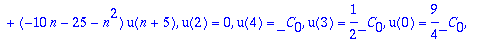and thus the first five zeroes of the Taylor expansion of the left-hand side of the functional equation conclude the proof.

Conclusion

These results are very good examples of the use of gfun : experiments first lead to conjecture a general form for the solution to a problem and then a completely different process leads to a proof. However, the apparent ease with which the problems treated here are solved using gfun hides the preliminary work which led to the form under which this approach could work. For example this approach does not seem to work for higher values of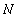, where similar results might exist.

Bibliography

 Arithmetic-Goemetric Means Revisited. Jonathan M. Borwein, Petr Lisonek and John A. Macdonald. MapleTech , 4-1 , pp. 20-27 (1997).